Say that you are a statistician and are asked to come up with a probability distribution for the current state of knowledge on some particular topic you know little about. (This, in Bayesian statistics, is known as choosing a suitable prior.) To do this, the safest bet is coming up with the least informative distribution via the principle of maximum entropy.

This principle is clearly explained by Jaynes (1968): consider a die which has been tossed a very large number of times $N$. We expect the average to be 3.5, that is, we expect a distribution where $P_n = \frac{1}{6}$ for each $n$, see the figure below.

begin
using AlgebraOfGraphics
using CairoMakie
using DataFrames
end
function plot_distribution(probabilities::Array)
np = mapping([1:6] => :n, [probabilities] => :P_n) * visual(BarPlot)
axis = (; xticks=1:6, limits=(nothing, (0, 1)), height=200)
draw(np; axis)
end;
plot_distribution([1/6, 1/6, 1/6, 1/6, 1/6, 1/6])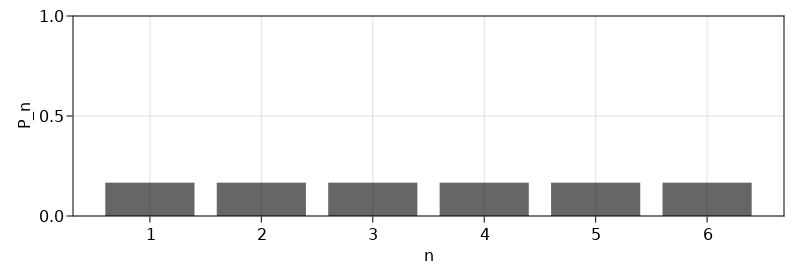Instead, we are told that the average is $4.5$. How likely is it for each number $n = 1,2, \ldots, 6$ to come up for the next toss?

Since we know that $P$ always sums to 1, we have

$$\sum_{n=1}^6 P_n = 1.$$

We also know that the average is $4.5$, that is,

$$\sum_{n=1}^6 n \cdot P_n = 4.5.$$

We could satisfy these constraints by choosing $P_4 = P_5 = \frac{1}{2}$.

plot_distribution([0, 0, 0, 0.5, 0.5, 0])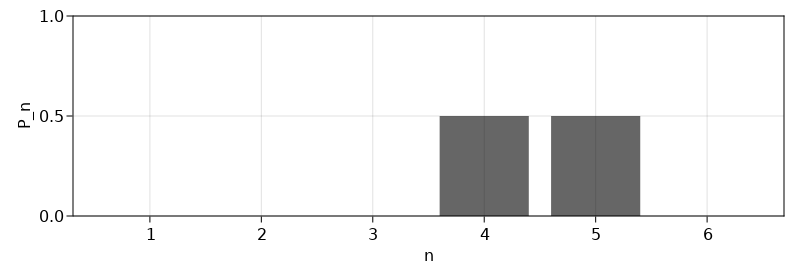This is unlikely to be the distribution for our data since it can be derived in relatively few ways, namely: by throwing only $4$ and $5$, and in such a way that the throws average to $4.5$. A more likely distribution would be

plot_distribution([0, 0, 1/4, 1/4, 1/4, 1/4])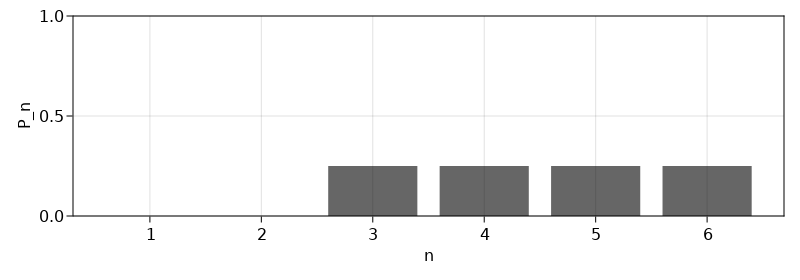This is still not the least informative distribution since it assumes $n = 1$ and $n = 2$ to be impossible events. Jaynes presents the straight line solution $P_n = (12n - 7)/210$,

plot_distribution([(12n - 7)/210 for n in 1:6])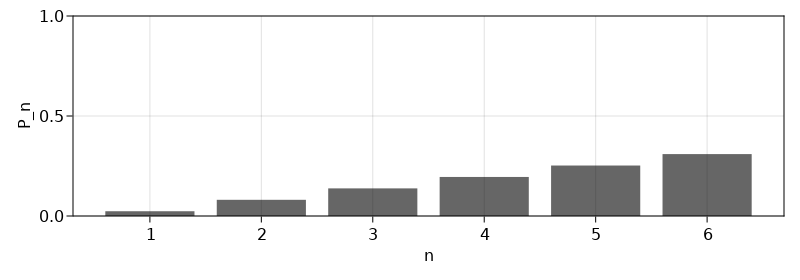This solution would also fail if the mean would have been higher, because then $P_0 = 0$ would occur again. The correct measure is the following information measure (Shannon, 1948) which is also known as information entropy,

$$S_I = - \sum_i p_i \log p_i.$$

We can find $p_i$ for $p_i = 1, 2, \ldots, 6$ by maximizing $S_I$ for given constraints. This problem, known as MaxEnt, is hard to solve manually since there are $6$ unknowns and various constraints. The solution can be approximated by rewriting it to a linear program.

Alternatively, analytic solutions exist for some subsets of this Shanon entropy maximization problem (Zabarankin and Uryasev, 2014) Here, we have that the mean is known (and nothing else), so the number of moments $m$ is $1$. Then, the maximum entropy distribution takes the form (Zabarankin and Uryasev, 2014; Eq. 5.1.7),

$$P_n = \frac{e^{\rho n}}{\sum_{n=1}^6 e^{\rho n}}, \: \text{ for } n = 1, 2, ..., 6.$$

This function satisfies $\sum_{n=1}^6 P_n = 1$ for any $\rho$. Now, we only have to find the $\rho$ for which the average is $4.5$. After some trial and error, you'll find that $\rho = 0.3715$ gives $\sum_{n=1}^6 n \cdot P_n \approx 4.501$.

plot_distribution([0.0543, 0.0787, 0.114, 0.165, 0.240, 0.348])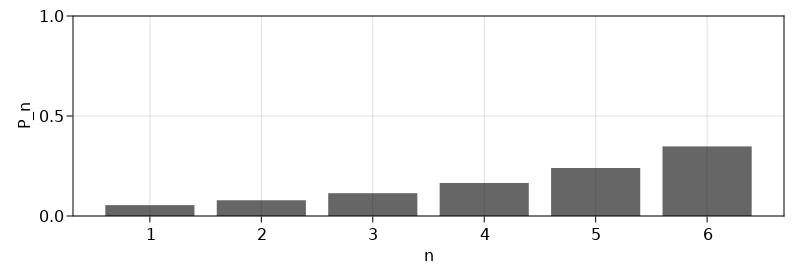This is the least informative distribution which satisfies the constraints. In other words, this is the distribution which can be obtained in the largest number of ways, given the constraints. For another example of maximum entropy distributions, see Chapter 10.1 of the book by McElreath (2020).

### Trial and error

p(k, rho) = exp(rho*k) / sum([exp(rho*1), exp(rho*2), exp(rho*3), exp(rho*4), exp(rho*5), exp(rho*6)])
p (generic function with 1 method)
function ps(rho)
values = map(k -> p(k, rho), 1:6)
@show values
sum_values = sum(values)
@show sum_values
average = sum([values*1, values*2, values*3, values*4, values*5, values*6])
@show average
Base.Text("""
values = $values sum_values:$sum_values
average = \$average
""")
end
ps (generic function with 1 method)
ps(0.4)
values = [0.04906874617024226, 0.0732019674190579, 0.1092045029116822, 0.16291397453728548, 0.24303909080562353, 0.36257171815610867]
sum_values: 1.0
average = 4.565367850857316

ps(0.34)
values = [0.060524771142131895, 0.08503413138555115, 0.11946849800579813, 0.16784697842149762, 0.23581620791666263, 0.3313094131283586]
sum_values: 1.0
average = 4.427323959970083

ps(0.3715)
values = [0.05426741458481562, 0.07868275019416264, 0.11408273685935422, 0.165409455277101, 0.2398284670256302, 0.3477291760589363]
sum_values: 1.0
average = 4.501036338141376


Built with Julia 1.7.2 and

AlgebraOfGraphics 0.6.4
CairoMakie 0.7.2
DataFrames 1.3.2